One mole of an ideal gas requires 207 J heat to raise the temperature by 10 K when heated at constant pressure. If the same gas is heated at constant volume to raise the temperature by the same 10 K, the heat required is

1.  198.7 J

2.  29 J

3.  215.3 J

4.  124 J

(Given the gas constant )

High Yielding Test Series + Question Bank - NEET 2020

Difficulty Level:

The expansion of an ideal gas of mass m at a constant pressure P is given by the straight line D. Then the expansion of the same ideal gas of mass 2m at a pressure P/ 2 is given by the straight line , where number on graphs indicate slope , is-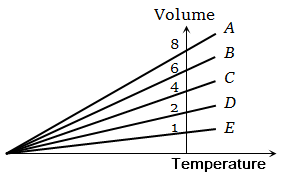1.  E

2.  C

3.  B

4.  A

High Yielding Test Series + Question Bank - NEET 2020

Difficulty Level:

An experiment is carried on a fixed amount of gas at different temperatures and at high pressure such that it deviates from the ideal gas behaviour. The variation of $\frac{\mathrm{PV}}{\mathrm{RT}}$ with P is shown in the diagram. The correct variation will correspond to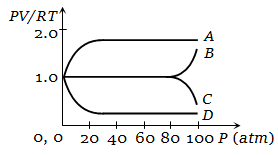1. Curve A

2. Curve B

3. Curve C

4. Curve D

High Yielding Test Series + Question Bank - NEET 2020

Difficulty Level:

The graph which represent the variation of mean kinetic energy of molecules with temperature t°C is

1.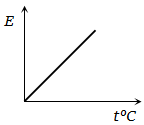2.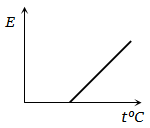3.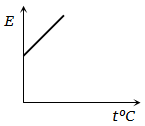4.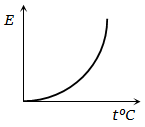High Yielding Test Series + Question Bank - NEET 2020

Difficulty Level:

The adjoining figure shows graph of pressure and volume of a gas at two temperatures ${\mathrm{T}}_{1}$ and ${\mathrm{T}}_{2}$. Which of the following interferences is correct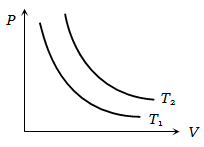1.  ${\mathrm{T}}_{1}>{\mathrm{T}}_{2}$

2. ${\mathrm{T}}_{1}={\mathrm{T}}_{2}$

3. ${\mathrm{T}}_{1}<{\mathrm{T}}_{2}$

4. No interference can be drawn

High Yielding Test Series + Question Bank - NEET 2020

Difficulty Level:

The expansion of until mass of a perfect gas at constant pressure is shown in the diagram. Here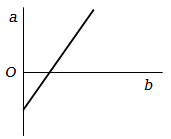1. a = volume, b = $°\mathrm{C}$ temperature

2. a = volume, b = $\mathrm{K}$ temperature

3. a = $°\mathrm{C}$ temperature, b = volume

4. a = $\mathrm{K}$ temperature, b = volume

High Yielding Test Series + Question Bank - NEET 2020

Difficulty Level:

An ideal gas is initially at temperature T and volume V. Its volume is increased by $∆\mathrm{V}$ due to an increase in temperature $∆\mathrm{T}$, pressure remaining constant. The quantity $\mathrm{\delta }=∆\mathrm{V}/\left(\mathrm{V}∆\mathrm{T}\right)$ varies with temperature as

1.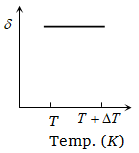2.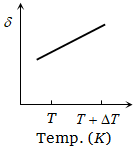3.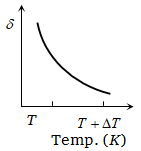4.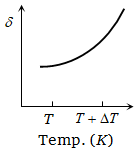High Yielding Test Series + Question Bank - NEET 2020

Difficulty Level:

Pressure versus temperature graph of an ideal gas of equal number of moles of different volumes are plotted as shown in figure. Choose the correct alternative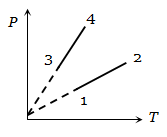1.

2.

3.

4.

High Yielding Test Series + Question Bank - NEET 2020

Difficulty Level:

Pressure versus temperature graph of an ideal gas is as shown in figure. Density of the gas at point A is ${\mathrm{\rho }}_{0}$ . Density at B will be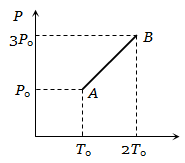1.  $\frac{3}{4}{\mathrm{\rho }}_{0}$

2.  $\frac{3}{2}{\mathrm{\rho }}_{0}$

3.  $\frac{4}{3}{\mathrm{\rho }}_{0}$

4.

High Yielding Test Series + Question Bank - NEET 2020

Difficulty Level:

The figure shows graphs of pressure versus density for an ideal gas at two temperatures ${\mathrm{T}}_{1}$ and ${\mathrm{T}}_{2}$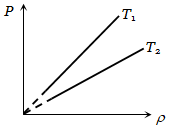1. ${\mathrm{T}}_{1}>{\mathrm{T}}_{2}$

2. ${\mathrm{T}}_{1}={\mathrm{T}}_{2}$

3. ${\mathrm{T}}_{1}<{\mathrm{T}}_{2}$

4. Nothing can be predicted